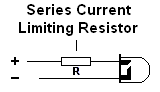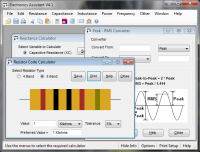# LED Series Resistor Calculator

These calculators give values for LED current limiting series resistors. The first calculator gives values based on standard LED types. This should only be used if the LED forward voltage and current are not known. The second calculator gives a more accurate value using these figures, which can be found in the suppliers catalogue. Preferred resistor values are also calculated; use the radio buttons on each calculator to select the preferred value series to use.#### Calculate Resistor Value for Common LED Types:

 Enter Supply Voltage: Volts Choose LED Type: Standard Low Current High Brightness Super Bright Ultra Bright Standard Blue Select preferred series (optional): E12 E24 E48 E96 E192 Resistor = ? Preferred Value = ?

#### Calculate Resistor Value from Vf and If:

 Enter Supply Voltage: Volts Enter LED Forward Voltage, Vf: Volts Enter LED Forward Current, If: Milliamps Select preferred series (optional): E12 E24 E48 E96 E192 Resistor = ? Preferred Value = ?

#### Formula:

R = (Vs - Vf)  / If

= (Supply Voltage - Forward Voltage) / Forward Current

If is in units of Amps, NOT Milliamps! (20mA = 0.02A)

For more details see the technical data section here.

# If you found this calulator useful you should try Electronics Assistant!• All the online calculators and more in a stand-alone application
• Converts Resistor & Inductor colour codes, calculates LED series resistors, capacitance units, series / parallel resistors & capacitors, frequency, reactance & more
• Calculation of nearest preferred resistor values with a choice of 5 series from E12 to E192
• Print & save calculation results